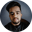Related Tags

solidity
loops
communitycreator

# What are loops in Solidity?Shubham Singh Kshatriya

We use loops when we want to perform an action repetitively. They make our code more organized and manageable.

Solidity is a language that supports the following loops used while creating smart contracts.

### 1. while loop

The while loop executes a block of code repeatedly, as long as the specified condition is true. When the condition becomes false, the loop will terminate.

If the condition is false at the start of the loop, it won’t execute the code.

### Syntax

while (condition) {
// body
}


### Example

In the following code snippet, we see the use of the while loop in Solidity:

pragma solidity ^0.5.0;

contract Example {
// declaring a state variable
uint i = 0;

// creating to function to use while loop
function whileLoop() public returns(uint) {
// creating a while loop
while (i < 5) {
i++;
}

// return i
return i;
}
}
while loop

### Explanation

• Line 3: We create a contract named Example.

• Line 5: We declare a state variable i.

• Line 8: We create a function, whileLoop. Inside this function, we create a while loop (line 10) to increment the value of i until the loop terminates.

• Line 15: We return the value of i.

### 2. do-while loop

The do-while loop is similar to the while loop with the difference that it checks the condition at the end of the loop.

Therefore, it executes a block of code at least once, even if the condition is false.

### Syntax

do {
// body
} while (condition);


### Example

In the following code snippet, we see the use of the do-while loop in Solidity:

pragma solidity ^0.5.0;

contract Example {
// declaring a state variable
uint i = 0;

// creating to function to use do-while loop
function doWhileLoop() public returns(uint) {
// creating a do-while loop
do {
i++;
} while (i < 5);

// return i
return i;
}
}
do-while loop

### Explanation

• Line 3: We create a contract named Example.

• Line 5: We declare a state variable, i.

• Line 8: We create a function doWhileLoop. Inside this function, we create a do-while loop (line 10) to increment the value of i until the loop terminates.

• Line 15: We return the value of i.

### 3. for loop

The for loop also repeatedly executes a block of code. It accepts the three following arguments separated by a semi-colon (;).

1. loop initialization: This initializes the iterator. It is executed only once.
2. loop condition: This checks whether or not the condition is true. If the condition is true, the body will be executed. If the condition is false, the loop will be terminated.
3. loop iteration: This updates the iterator’s value. After updating the value, it will recheck the loop condition.

### Syntax

for (initialization; condition; iteration) {
// body
}


### Example

In the following code snippet, we see the use of the for loop in Solidity.

pragma solidity ^0.5.0;

contract Example {
// declaring a state variable
uint i = 0;

// creating to function to use for loop
function forLoop() public returns(uint) {
// creating a for loop
for (uint j = 0; j < 5; j++) {
i++;
}

// return i
return i;
}
}
for loop

### Explanation

• Line 3: We create a contract named Example.

• Line 5: We declare a state variable, i.

• Line 8: We create a function, forLoop. Inside this, we create a for loop (line 10) to increment the value of i until the loop terminates.

• Line 15: We return the value of i.

RELATED TAGS

solidity
loops
communitycreator

CONTRIBUTORShubham Singh Kshatriya
RELATED COURSES

View all Courses

Keep Exploring

Learn in-demand tech skills in half the time